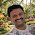## Wednesday, August 3, 2011

### How to create a prismatic prescription through decentration!

It is one of the most useful techniques,

“Power x Decentration(in centimeters) = Prism”

∆ =  100 x X x D
“∆“ is the prism, in prism diopters, which equals “ D”, the back vertex power times “X”, the decentration times “100”, which converts from meters to centimeters. Its called PRENTICE’S RULE
Normally, eyeglasses are fitted with the optical center of the lens directly in front of the eye. If the lens is fit off-center, image displacement can occur due to induced prism. The higher the power or the further the lens is fit off-center, the higher is the induced prismatic effect.

#### Induced Prism

To better understand why there is induced prism, the cross-section of a plus lens can be likened to two prisms base-to-base, as the lens is thicker in the middle and thinner at the edges. Likewise, a minus lens can be likened to two prisms apex-to-apex, thinner in the middle and thicker at the edges. This induced prism can actually be used to the advantage of the lab when prism is called for in a prescription. If the lens power is sufficient, to induce the prescribed prism, the lens can simply be cut off-center to achieve the required results. This is known as prism by decentration. If the power is insufficient(if less than 1D), however, the prism must be cut into the surface of the lens. A simple equation can be used to calculate the prism induced by decentration. Prentice’s rule states that prism in diopters (Δ) is equal to the decentration distance (c) in centimeters multiplied by the lens power (D). We can create a prismatic prescription through decentration using a finished uncut, thereby avoiding the time and expense of having to grind the Rx from a semi-finished blank.

Decentration = Prism * 10 / Power
This gives you the decentration in mm.

If (power<0)
Base In Prism, decentration = +
Base Out Prism, decentration = -
Base Up Prism, decentration = +
Base Down Prism, decentration = -

If (power>0)
Base In Prism, decentration = -
Base Out Prism, decentration = +
Base Up Prism, decentration = -
Base Down Prism, decentration = +

Example: -5.00sph lens need with 2.5∆ base in
Decentration = 2.5 x 10 / -5 = 5mm
As this is base In prism and the power is minus(<0), so that you have to add 5mm to the mono PD to get the required prism(2.5∆)
Important thing to remember: Prentice’s Rule breaks down for ophthalmic lens powers of less than about ± 1.00diopter.

Ref: laramyk, optiboard, moamember

1.Simply put, if you have a plus lens decentration goes with it. While in a minus lens, decentration goes against it.

1.Exactly said

2.Nice description how to use sign when doing numerical

3.Nice description how to use sign when doing numerical

4.Hey if prescription is only hv cylinder power.. Then?

5.Hey if prescription is only hv cylinder power.. Then?